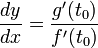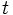# Parametric derivative

The parametric derivative$dy/dx$ for a parametric curve$x = f(t), y = g(t)$ at a point$t = t_0$ is given as:$\! \frac{dy}{dx} = \frac{g'(t_0)}{f'(t_0)}$
As a general function of$t$, the parametric derivative$dy/dx$ is defined as$g'(t)/f'(t)$.
MORE ON THE WAY THIS DEFINITION OR FACT IS PRESENTED: We first present the version that deals with a specific point (typically with a$\{ \}_0$ subscript) in the domain of the relevant functions, and then discuss the version that deals with a point that is free to move in the domain, by dropping the subscript. Why do we do this?